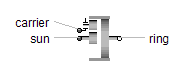DC Permanent Magnet - MapleSim Help

DC Permanent Magnet

Permanent magnet DC machineDescription The quasistationary DC Permanent Magnet component models a DC Machine with permanent magnets. This model is fully compatible with the regular DC Permanent Magnet model; the only difference is that electrical transients are neglected.Variables

 Name Units Description Modelica ID $\mathrm{phiMechanical}$ $\mathrm{rad}$ Mechanical angle of rotor against stator phiMechanical $\mathrm{wMechanical}$ $\frac{\mathrm{rad}}{s}$ Mechanical angular velocity of rotor against stator wMechanical $\mathrm{tauElectrical}$ $Nm$ Electromagnetic torque tauElectrical $\mathrm{tauShaft}$ $Nm$ Shaft torque tauShaft $\mathrm{inertiaRotor}$ inertiaRotor $\mathrm{inertiaStator}$ inertiaStator $\mathrm{fixed}$ fixed $\mathrm{friction}$ friction $\mathrm{va}$ $V$ Armature voltage va $\mathrm{ia}$ $A$ Armature current ia $\mathrm{ra}$ ra $\mathrm{la}$ la $\mathrm{brush}$ brush $\mathrm{core}$ core $\mathrm{strayLoad}$ strayLoad $\mathrm{thermalAmbient}$ thermalAmbient $\mathrm{airGapDC}$ airGapDC $\mathrm{eGround}$ eGround $\mathrm{ie}$ ie

 $\mathrm{flange}\cdot \mathrm{\phi }-\mathrm{internalSupport}\cdot \mathrm{\phi }$

 $\mathrm{inertiaRotor}\cdot {\mathrm{flange}}_{a}\cdot \mathrm{\tau }$Connections

 Name Description Modelica ID $\mathrm{flange}$ Shaft flange ${\mathrm{pin}}_{\mathrm{an}}$ Negative armature pin pin_an ${\mathrm{pin}}_{\mathrm{ap}}$ Positive armature pin pin_ap $\mathrm{support}$ Support at which the reaction torque is acting support $\mathrm{thermalPort}$ Optional thermal port thermalPortParametersGeneral Parameters

 Name Default Units Description Modelica ID ${J}_{r}$ $\mathrm{kg}{m}^{2}$ Rotor moment of inertia Jr ${J}_{s}$ $\mathrm{kg}{m}^{2}$ Stator moment of inertia Js ${T}_{a,\mathrm{oper}}$ $K$ Operational armature temperature TaOperational Use Support Flange $\mathrm{false}$ True (checked) means enable stator support useSupport Use Thermal Port $\mathrm{false}$ True (checked) means thermal port is enabled useThermalPortBrush Losses

 Name Default Units Description Modelica ID $V$ $0$ $V$ Total voltage drop of brushes, if ${i}_{\mathrm{brush}}>{I}_{\mathrm{linear}}$ V ${I}_{\mathrm{linear}}$ $0.01{I}_{{a}_{\mathrm{nom}}}$ $A$ Current indicating linear voltage region of brush voltage drop ILinear

See Brush for details of the brush loss model.Core Losses

 Name Default Units Description Modelica ID ${P}_{\mathrm{ref}}$ $0$ $W$ Reference rotor core losses PRef ${V}_{\mathrm{ref}}$ ${V}_{\mathrm{nom}}$ $V$ Reference RMS voltage VRef ${\omega }_{\mathrm{ref}}$ ${\omega }_{\mathrm{nom}}$ $\frac{\mathrm{rad}}{s}$ Reference angular velocity wRef

See Core for details of the core loss model.Friction Losses

 Name Default Units Description Modelica ID ${P}_{\mathrm{ref}}$ $0$ $W$ Reference friction losses PRef ${\omega }_{\mathrm{ref}}$ ${\omega }_{\mathrm{nom}}$ $\frac{\mathrm{rad}}{s}$ Reference angular velocity wRef ${p}_{\omega }$ $2$ $V$ Exponent of friction power_w

See Friction for details of the friction loss model.Name Default Units Description Modelica ID ${I}_{\mathrm{ref}}$ ${I}_{{a}_{\mathrm{nom}}}$ $W$ Reference RMS current IRef ${P}_{\mathrm{ref}}$ $0$ $W$ Reference friction losses PRef ${\omega }_{\mathrm{ref}}$ ${\omega }_{\mathrm{nom}}$ $\frac{\mathrm{rad}}{s}$ Reference angular velocity wRef ${p}_{\omega }$ $1$ $V$ Exponent of stray load loss power_wNominal Parameters Parameters

 Name Default Units Description Modelica ID ${I}_{a,\mathrm{nom}}$ $A$ Nominal armature current IaNominal ${\mathrm{\omega }}_{\mathrm{nom}}$ $\frac{\mathrm{rad}}{s}$ Nominal rotational speed wNominal ${T}_{a,\mathrm{nom}}$ $K$ Nominal armature temperature TaNominal ${V}_{a,\mathrm{nom}}$ $V$ Nominal armature voltage VaNominalNominal Resistances And Inductances Parameters

 Name Default Units Description Modelica ID ${\mathrm{\alpha }}_{a}$ $\frac{1}{K}$ Temperature coefficient of armature resistance alpha20a ${L}_{a}$ $H$ Armature inductance La ${R}_{a}$ $\mathrm{\Omega }$ Warm armature resistance Ra ${T}_{a,\mathrm{ref}}$ $K$ Reference temperature of armature resistance TaRefModelica Standard Library The component described in this topic is from the Modelica Standard Library. To view the original documentation, which includes author and copyright information, click here.